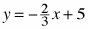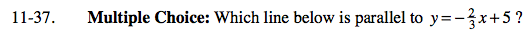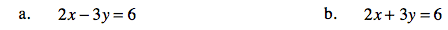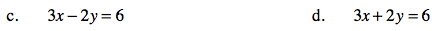Home > AC > Chapter 11 > Lesson 11.1.3 > Problem11-37

11-37.
1. Multiple Choice: Which line below is parallel to? Homework Help ✎

1. 2x − 3y = 6

2. 2x + 3y = 6

3. 3x − 2y = 6

4. 3x + 2y = 6Rearrange the equations into slope intercept form. Remember that parallel lines have equal slopes.

B